Group Replacement Policy

The replacement of all equipment at any predetermined interval is established under this policy. In this,the machinery are replaced irrespective of the fact that they have stopped functioning or not. Also when the faulty equipment are changed, others having even the minimal service period is also changed. The ideal instance where this policy is appropriate are those instances where the machinery have similar low cost and also the probability to get broken down at nearly same time. In those cases, replacement of equipment at predetermined intervals is an economical investment.

The variables can be depicted as,

n = total number of machinery in a system

C (f) = replacement cost of one failed equipmen

N (t) = number of equipment which stops working during ‘t’ period

C (g) = group replacement cost

C (t) = group replacement cost after the determined time ‘t’

C (t) / t = average costing per unit time

C (t) = n C (g)+ C (f) (n1 + n2 + …. +nt-1)

F (t) = Average cost per unit time, which is = C (t)/t = n Cg + Cf(n1 + n2 + …. + nt-1)/t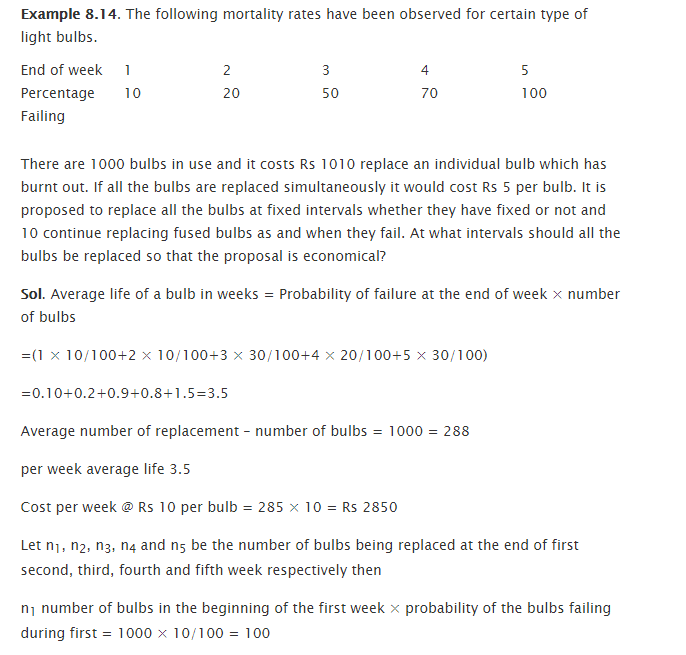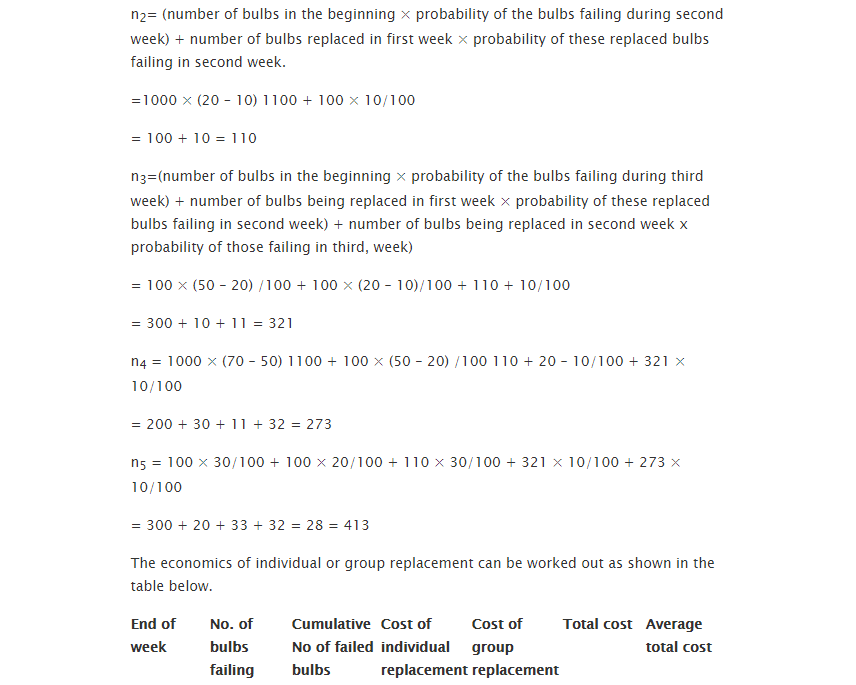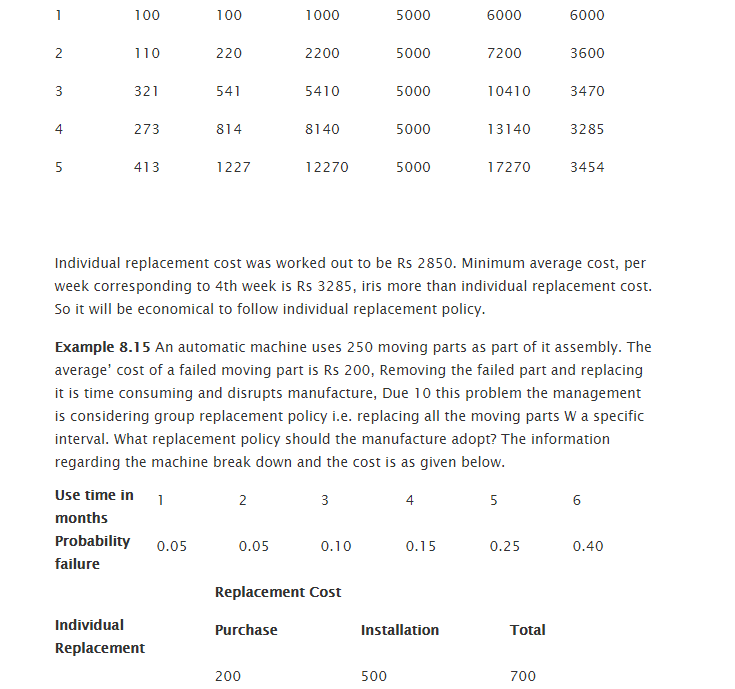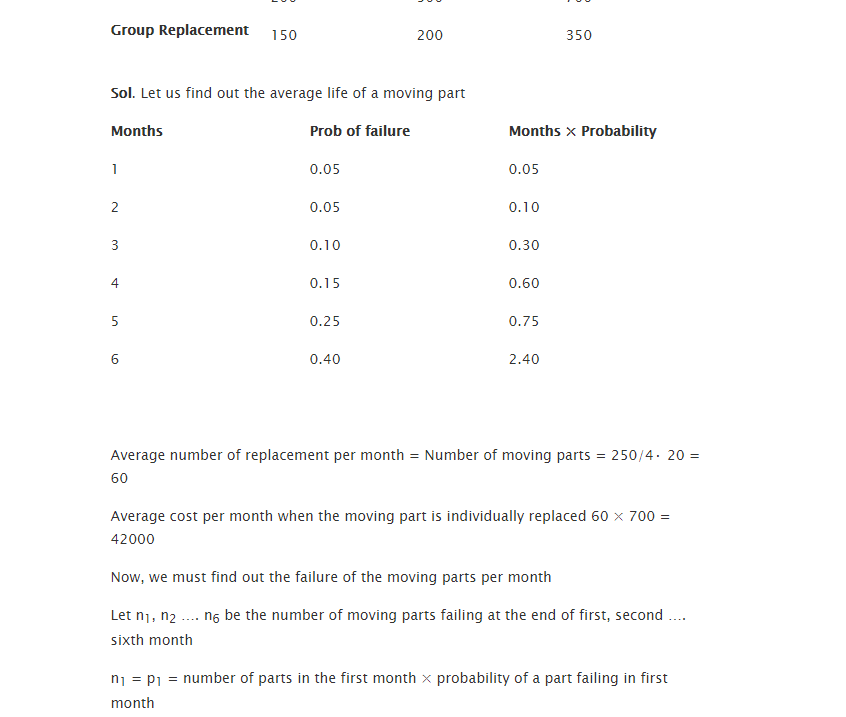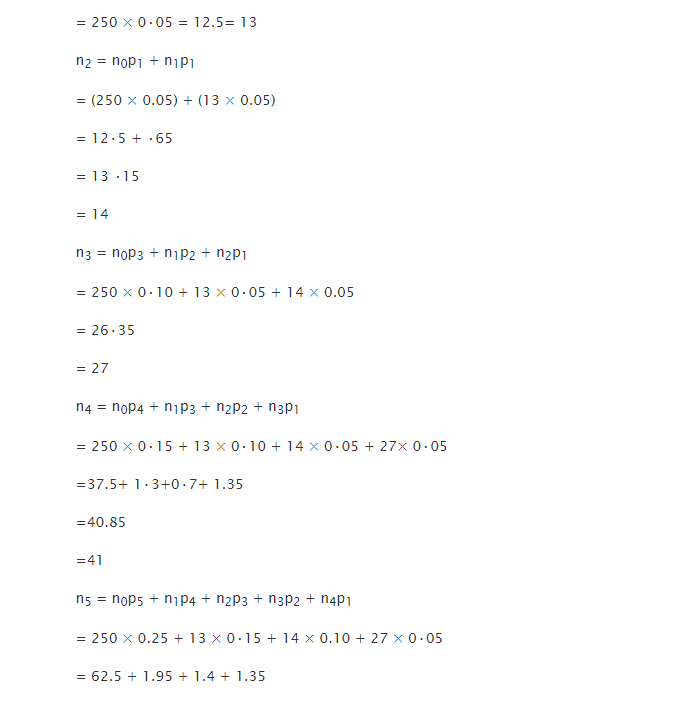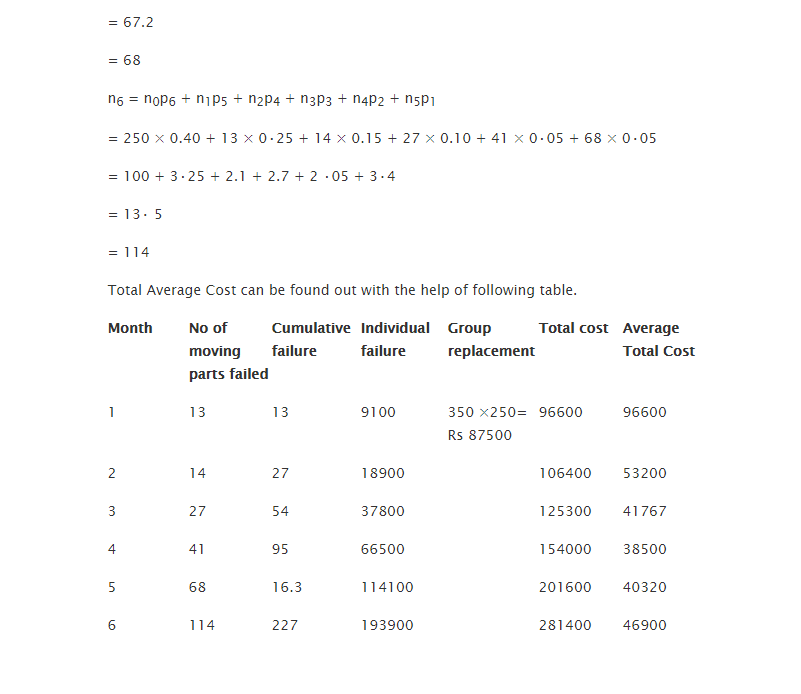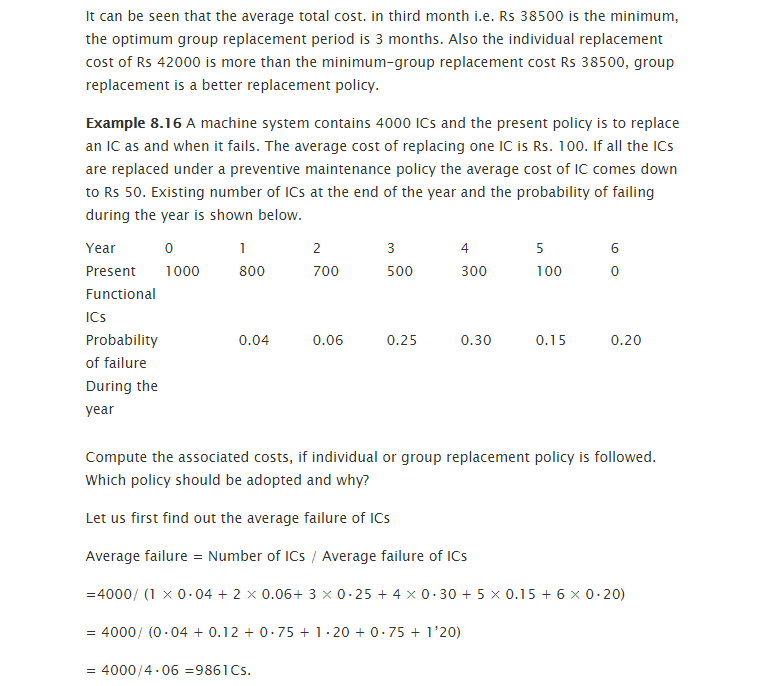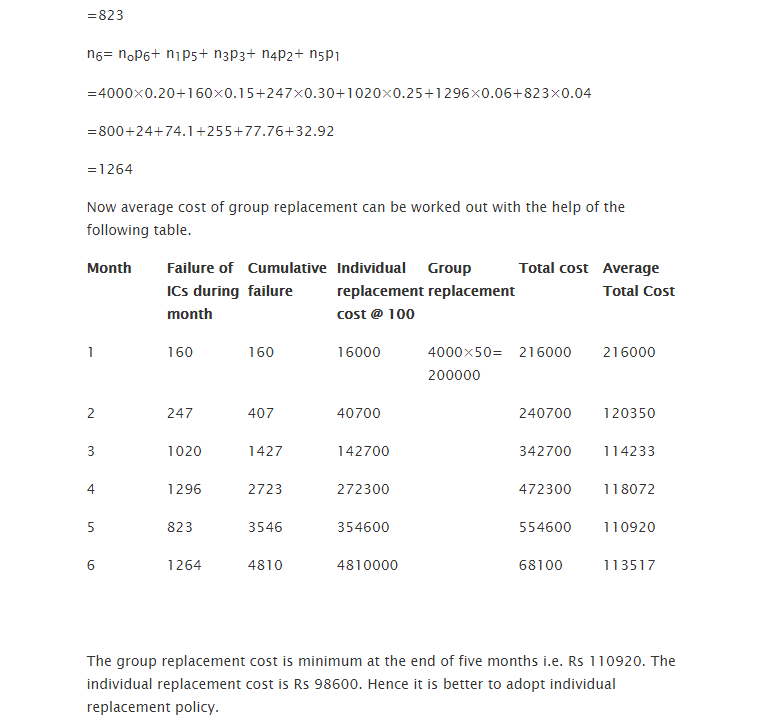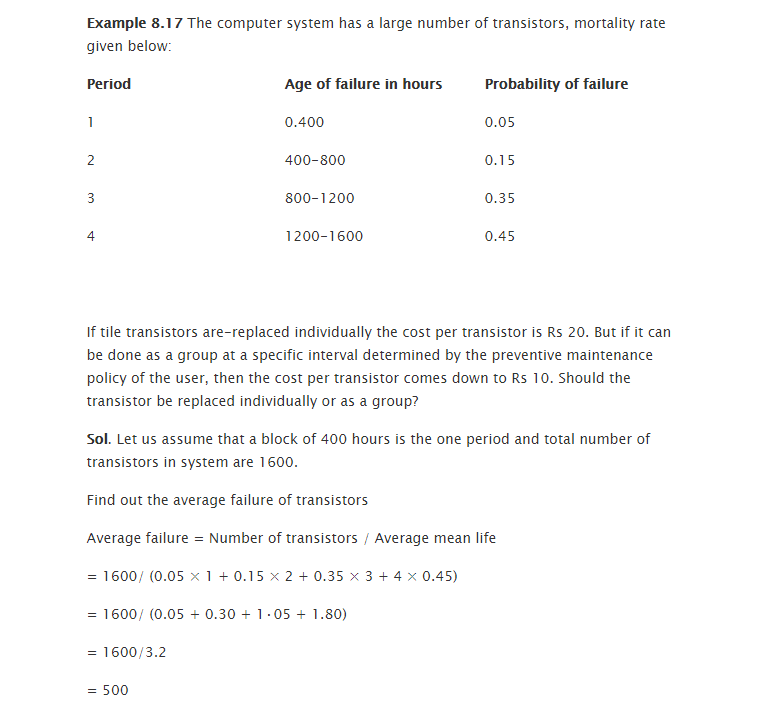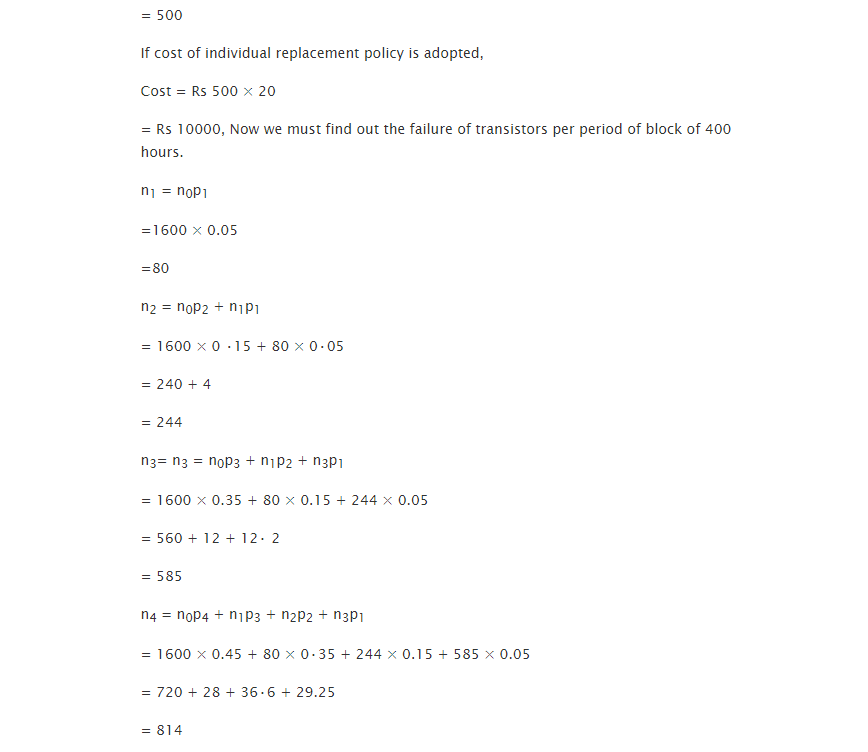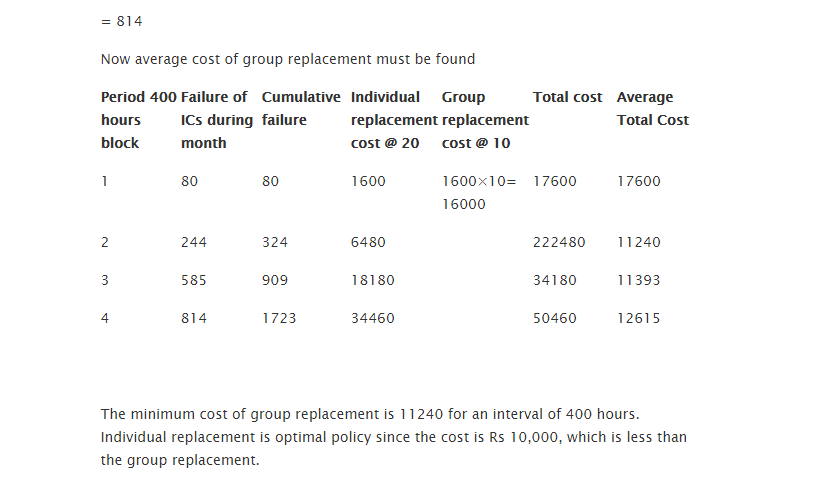### Customer Reviews